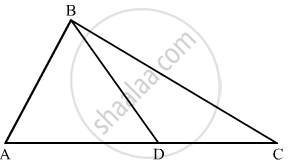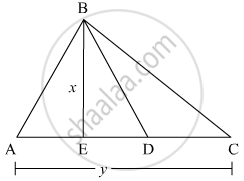# In the given figure, the ratio AD to DC is 3 to 2. If the area of Δ ABC is 40 cm2, what is the area of Δ BDC? - Mathematics

In the given figure, the ratio AD to DC is 3 to 2. If the area of Δ ABC is 40 cm2, what is the area of Δ BDC?#### Solution

Area of triangle ABC is given 40 cm2.Also AD : DC = 3:2

We are asked to find the area of the triangle BDC

Let us take BE perpendicular to base AC in triangle ABC.

We assume AC equal to y and BE equal to x in triangle ABC

Area of triangle ABC, say A is given by

A = 1/2  ( Base × Height)

40 = 1/2 (AC xx BE )

40 = 1/2 ( y xx x)

xy = 40 xx 2

xy = 80

We are given the ratio between AD to DC equal to 3:2

So,

AD = 3/5 y

DC = 2/5 y

In triangle BDC, we take BE as the height of the triangle

Area of triangle BDC, say A1 is given by

A_1 = 1/2 ( Base × Height )

A_1 = 1/2 ( DC xx BE )

A_1 = 1/2 (2/5y xx x)

A_1 = 1/5 xy

A_1 = 1/5 xx 80 ( as xy = 80 )

A_1 = 16   cm^2

Concept: Application of Heron’s Formula in Finding Areas of Quadrilaterals
Is there an error in this question or solution?

#### APPEARS IN

RD Sharma Mathematics for Class 9
Chapter 17 Heron’s Formula
Exercise 17.4 | Q 12 | Page 25

Share